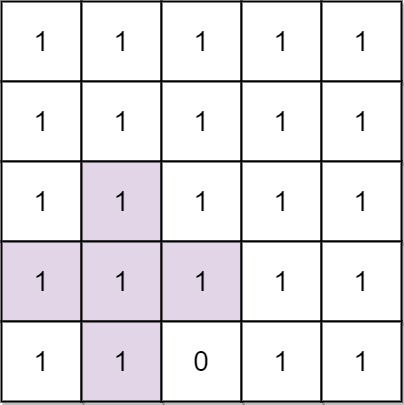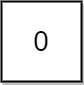## Algorithm

Problem Name: 764. Largest Plus Sign

You are given an integer `n`. You have an `n x n` binary grid `grid` with all values initially `1`'s except for some indices given in the array `mines`. The `ith` element of the array `mines` is defined as `mines[i] = [xi, yi]` where `grid[xi][yi] == 0`.

Return the order of the largest axis-aligned plus sign of 1's contained in `grid`. If there is none, return `0`.

An axis-aligned plus sign of `1`'s of order `k` has some center `grid[r][c] == 1` along with four arms of length `k - 1` going up, down, left, and right, and made of `1`'s. Note that there could be `0`'s or `1`'s beyond the arms of the plus sign, only the relevant area of the plus sign is checked for `1`'s.

Example 1:```Input: n = 5, mines = [[4,2]]
Output: 2
Explanation: In the above grid, the largest plus sign can only be of order 2. One of them is shown.
```

Example 2:```Input: n = 1, mines = [[0,0]]
Output: 0
Explanation: There is no plus sign, so return 0.
```

Constraints:

• `1 <= n <= 500`
• `1 <= mines.length <= 5000`
• `0 <= xi, yi < n`
• All the pairs `(xi, yi)` are unique.

## Code Examples

### #1 Code Example with Javascript Programming

```Code - Javascript Programming```

``````
const orderOfLargestPlusSign = function (N, mines) {
const dp = [...Array(N)].map((_) => Array(N).fill(N))
mines.map((m) => {
dp[m][m] = 0
})
for (let i = 0; i < N; i++) {
for (let j = 0, k = N - 1, l = (r = u = d = 0); j < N; j++, k--) {
dp[i][j] = Math.min(dp[i][j], (l = dp[i][j] == 0 ? 0 : l + 1))
dp[i][k] = Math.min(dp[i][k], (r = dp[i][k] == 0 ? 0 : r + 1))
dp[j][i] = Math.min(dp[j][i], (d = dp[j][i] == 0 ? 0 : d + 1))
dp[k][i] = Math.min(dp[k][i], (u = dp[k][i] == 0 ? 0 : u + 1))
}
}
let max = 0
for (let i = 0; i < N; i++) {
for (let j = 0; j < N; j++) {
max = Math.max(dp[i][j], max)
}
}
return max
}
``````
Copy The Code &

Input

cmd
n = 5, mines = [[4,2]]

Output

cmd
2

### #2 Code Example with Python Programming

```Code - Python Programming```

``````
class Solution:
def orderOfLargestPlusSign(self, N, mines):
#up, left, down, right
dp, res, mines = [[[0, 0, 0, 0] for j in range(N)] for i in range(N)], 0, {(i, j) for i, j in mines}
for i in range(N):
for j in range(N):
if (i, j) not in mines:
try:
dp[i][j] = dp[i - 1][j] + 1
except:
dp[i][j] = 1
try:
dp[i][j] = dp[i][j - 1] + 1
except:
dp[i][j] = 1
for i in range(N - 1, -1, -1):
for j in range(N - 1, -1, -1):
if (i, j) not in mines:
try:
dp[i][j] = dp[i + 1][j] + 1
except:
dp[i][j] = 1
try:
dp[i][j] = dp[i][j + 1] + 1
except:
dp[i][j] = 1
res = max(res, min(dp[i][j]))
return res
``````
Copy The Code &

Input

cmd
n = 5, mines = [[4,2]]

Output

cmd
2